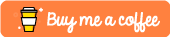#Implementing The Insertion Sort Algorithm in Python

This tutorial was built using Python 3.6

In this tutorial we are going to be taking a look at the insertion sorting algorithm and how it works as well as how you can implement this algorithm in the Python programming language.

# Insertion Sorting

So the insertion sorting algorithm is a well known sorting algorithm that can sort an unsorted array in a worst case time of `O(N^2)` time. It works by iterating through an array and sorting elements in a linear fashion.

• The algorithm starts at element `0` in an array and considers element sorted.
• It then looks at the first element to the right of our sorted array that just contains our `0` position element
• It then inserts this unsorted element in to it’s correct location.
• Our sorted array now has 2 elements
• The algorithm proceed to insert the next element into the correct position in our sorted array until the entire list has been sorted.

# Implementing it in Python

Implementing it in Python can be done like so:

``````# Insertion Sort In Python
#
# Performance Complexity = O(n^2)
# Space Complexity = O(n)

def insertionSort(my_list):
# for every element in our array
for index in range(1, len(my_list)):
current = my_list[index]
position = index

while position > 0 and my_list[position-1] > current:
print("Swapped {} for {}".format(my_list[position], my_list[position-1]))
my_list[position] = my_list[position-1]
print(my_list)
position -= 1

my_list[position] = current

return my_list

my_list = [8,2,1,3,5,4]

print(insertionSort(my_list))``````

When executed this should give you the following output:

`````` \$ python3.6 insertionSort.py
Swapped 2 for 8
[8, 8, 1, 3, 5, 4]
Swapped 1 for 8
[2, 8, 8, 3, 5, 4]
Swapped 8 for 2
[2, 2, 8, 3, 5, 4]
Swapped 3 for 8
[1, 2, 8, 8, 5, 4]
Swapped 5 for 8
[1, 2, 3, 8, 8, 4]
Swapped 4 for 8
[1, 2, 3, 5, 8, 8]
Swapped 8 for 5
[1, 2, 3, 5, 5, 8]
[1, 2, 3, 4, 5, 8]``````

# Conclusion

If you found this tutorial useful or require further assistance then please let me know in the comments section below!